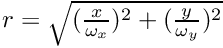BornAgain  1.19.0 Simulate and fit neutron and x-ray scattering at grazing incidence
Fourier-space decay functions

## Classes

class  FTDecayFunction1DCauchy
One-dimensional Cauchy decay function in reciprocal space; corresponds to exp(-|x|/decay_length) in real space. More...

class  FTDecayFunction1DGauss
One-dimensional Gauss decay function in reciprocal space; corresponds to exp[-x^2/(2*decay_length^2)] in real space. More...

class  FTDecayFunction1DTriangle
One-dimensional triangle decay function in reciprocal space; corresponds to 1-|x|/decay_length if |x|<decay_length (and 0 otherwise) in real space. More...

class  FTDecayFunction1DVoigt
One-dimensional pseudo-Voigt decay function in reciprocal space; corresponds to eta*Gauss + (1-eta)*Cauchy. More...

class  FTDecayFunction2DCauchy
Two-dimensional Cauchy decay function in reciprocal space; corresponds to exp(-r) in real space, with. More...

class  FTDecayFunction2DGauss
Two-dimensional Gauss decay function in reciprocal space; corresponds to exp(-r^2/2) in real space, with. More...

class  FTDecayFunction2DVoigt
Two-dimensional pseudo-Voigt decay function in reciprocal space; corresponds to eta*Gauss + (1-eta)*Cauchy. More...